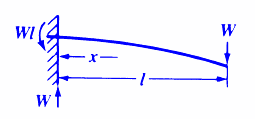Beam Bending Equations Calculator
Cantilevered Beam with One Load Applied at End

Beam Deflection and Stress Formula and Calculators

To see the equations and applicable units behind this calculator see:
Equations for Cantilevered Beam with One Load Applied at End Calculator

This structural calculator will determine the stress, deflections, area moment of inertia, and section modulus for a Cantilevered Beam with One Load Applied at End.Design Variables Modulus of Elasticity lbs/in2 (N/mm2) E = Moment of Inertia in4 (mm4) I = Load lbs (N) W = Distance in. (mm) l = Distance in. (mm) x = Distance to nuetral axis/plane in. (mm) z = Results Stress at specific point lbs/in2 (N/mm2) s = Stress at the support lbs/in2 (N/mm2) s = Deflection at specified point in. (mm) y = Deflection at unsupported end in. (mm) y = Section Modulus in3 (mm3) Z =

Automatic recalculation© Copyright 2000 - 2019, by Engineers Edge, LLC www.engineersedge.comMembership Register | LoginHomeEngineering Book StoreEngineering ForumExcel App. DownloadsOnline Books & ManualsEngineering NewsEngineering VideosEngineering CalculatorsEngineering ToolboxGD&T Training Geometric Dimensioning TolerancingDFM DFA TrainingTraining Online EngineeringAdvertising CenterCopyright Notice